Strategies to Add & Subtract within 20 | K, 1st & 2nd Grade
1%
It was processed successfully!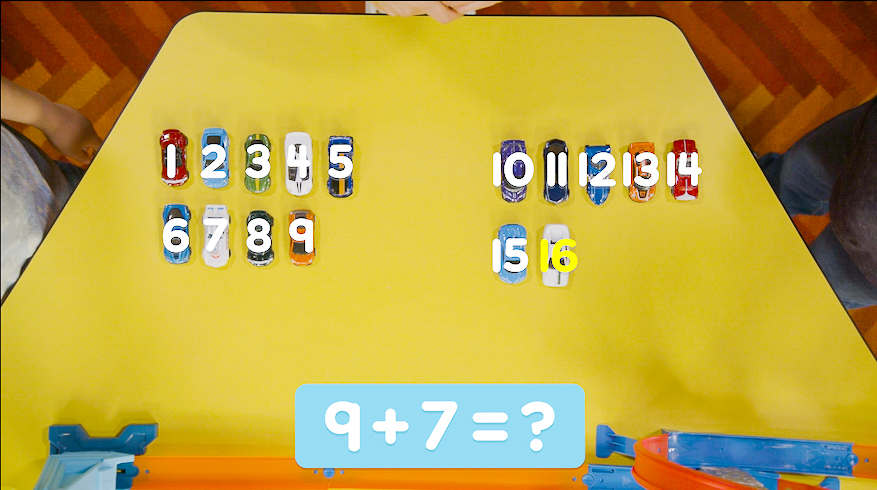WHAT IS A STRATEGY?

A strategy is a way you can tackle a problem. In math, there are often many strategies you use to solve a problem. These strategies can all give you the correct answer! Just pick the one that makes the most sense to you.

To better understand strategies…

WHAT IS A STRATEGY?. A strategy is a way you can tackle a problem. In math, there are often many strategies you use to solve a problem. These strategies can all give you the correct answer! Just pick the one that makes the most sense to you. To better understand strategies…

## LET’S BREAK IT DOWN!

### Counting all to find a sum.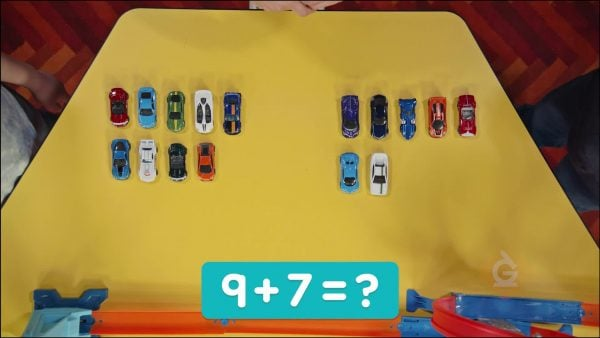You have 9 toy cars and 7 toy cars. How many cars do you have in all? 9 + 7 = ? You can count all of the cars to find the sum. 1, 2, 3, 4, 5, 6, 7, 8, 9, 10, 11, 12, 13, 14, 15, 16. There are 16 cars in all.

Counting all to find a sum. You have 9 toy cars and 7 toy cars. How many cars do you have in all? 9 + 7 = ? You can count all of the cars to find the sum. 1, 2, 3, 4, 5, 6, 7, 8, 9, 10, 11, 12, 13, 14, 15, 16. There are 16 cars in all.

### Make a ten to find how many cars.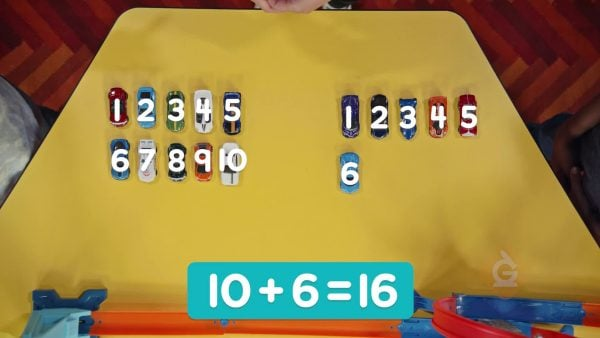Counting all of the cars will get you the correct answer but it takes a long time. Especially when numbers get bigger! You can make a ten to find how many in all. 9 + 1 = 10, so take 1 from 7 and add it to the 9. That leaves 6 left. So, 9 + 7 = 10 + 6. There are 16 cars in all.

Make a ten to find how many cars. Counting all of the cars will get you the correct answer but it takes a long time. Especially when numbers get bigger! You can make a ten to find how many in all. 9 + 1 = 10, so take 1 from 7 and add it to the 9. That leaves 6 left. So, 9 + 7 = 10 + 6. There are 16 cars in all.

### Use doubles facts to add spots.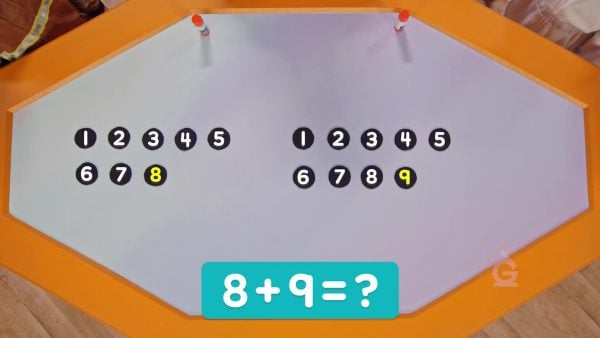A Dalmatian has 8 spots and 9 spots. How many spots in all? 8 and 9 are close to a double. 8 + 8 = 16. 9 is 1 more than 8. So 8 + 9 is the same as 8 + 8 + 1, or 16 + 1 = 17. There are 17 spots in all.

Use doubles facts to add spots. A Dalmatian has 8 spots and 9 spots. How many spots in all? 8 and 9 are close to a double. 8 + 8 = 16. 9 is 1 more than 8. So 8 + 9 is the same as 8 + 8 + 1, or 16 + 1 = 17. There are 17 spots in all.

### Count to compare cherries.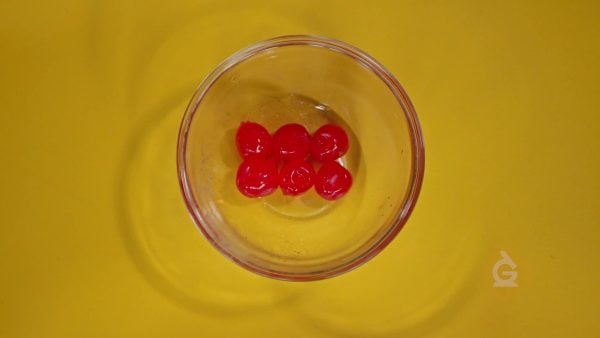A jar has 15 cherries. You use 9 cherries. How many cherries are left? You can count back from 15 to 9 find the difference. 14, 13, 12, 11, 10, 9. You can also count on to find a difference. Count on from 9 to 15. 10, 11, 12, 13, 14, 15. You counted 6 numbers both ways. There are 6 cherries left.

Count to compare cherries. A jar has 15 cherries. You use 9 cherries. How many cherries are left? You can count back from 15 to 9 find the difference. 14, 13, 12, 11, 10, 9. You can also count on to find a difference. Count on from 9 to 15. 10, 11, 12, 13, 14, 15. You counted 6 numbers both ways. There are 6 cherries left.

### Make a ten to subtract.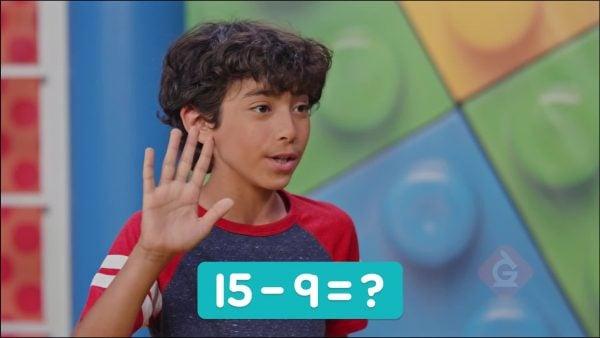You can make a ten to subtract. Start with 15 cherries. 15 is 10 and 5 more, so break 9 into 5 and 4. Subtract 5: 15 – 5 = 10. Then subtract 4 more. 10 – 4 = 6. So, 15 – 9 = 6.

Make a ten to subtract. You can make a ten to subtract. Start with 15 cherries. 15 is 10 and 5 more, so break 9 into 5 and 4. Subtract 5: 15 – 5 = 10. Then subtract 4 more. 10 – 4 = 6. So, 15 – 9 = 6.

## STRATEGIES TO ADD & SUBTRACT WITHIN 20 VOCABULARY

Finding the total, or sum, by combining two or more numbers.
Sum
Subtraction
Taking one number away from another.
Difference
The result of subtracting.
Decompose
Breaking a number into two or more parts.
When adding two numbers, break one number into two parts, so that one part added to the first number makes 10.
When adding two numbers, break one number into two parts so that two of the numbers make a doubles fact.
When subtracting two numbers, count on from the smaller number to the bigger number. How many you counted on is the difference.
When subtracting two numbers, count back from the bigger number the amount given by the smaller number. Where you stop counting is the difference.

## STRATEGIES TO ADD & SUBTRACT WITHIN 20 DISCUSSION QUESTIONS

### What strategy would you use to find 6 + 7?

Answers will vary. Students may make a 10 by adding 6 + 4 + 3. Students may use a doubles fact: 6 + 6 + 1.

### What strategy would you use to find 8 + 4?

Answers will vary. Students may make a 10 by adding 8 + 2 + 2. Students may use doubles facts and add 4 + 4 + 4.

### How do you decide which addition strategy to use?

Answers will vary. Students may say they always use one strategy or that they choose the doubles strategy if the addends are the same or 1 apart.

### What strategy would you use to find 14 – 7?

Answers will vary. Students may describe a counting back strategy: 14 – 4 = 10, 10 – 3 = 7. They may count on 7 + 3 = 10, 10 + 4 = 14. 3 + 4 = 7. Students may also remember that 14 and 7 are part of a doubles fact, so the difference must be 7.

### How do you decide which subtraction strategy to use?

Answers will vary. Students may say they always use one strategy or that they choose the count on strategy when they have to 'cross 10' when subtracting.
X

## Success

We’ve sent you an email with instructions how to reset your password.
Ok